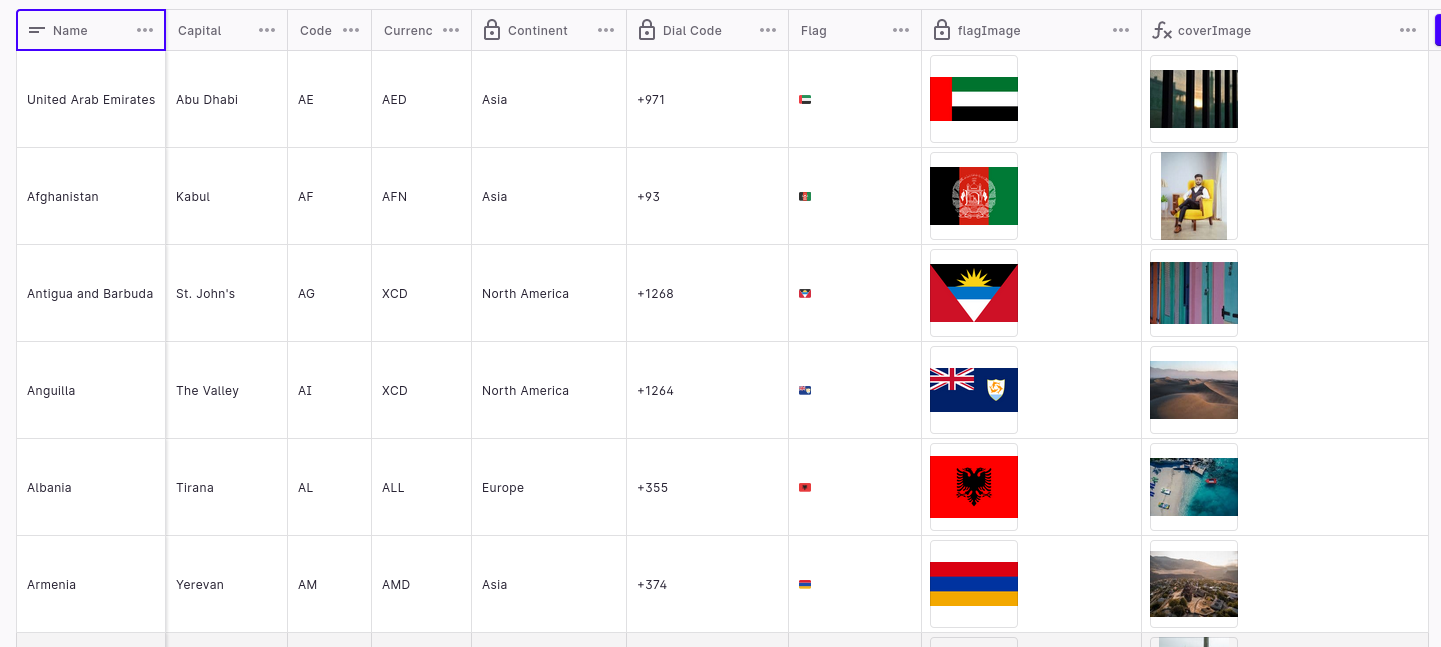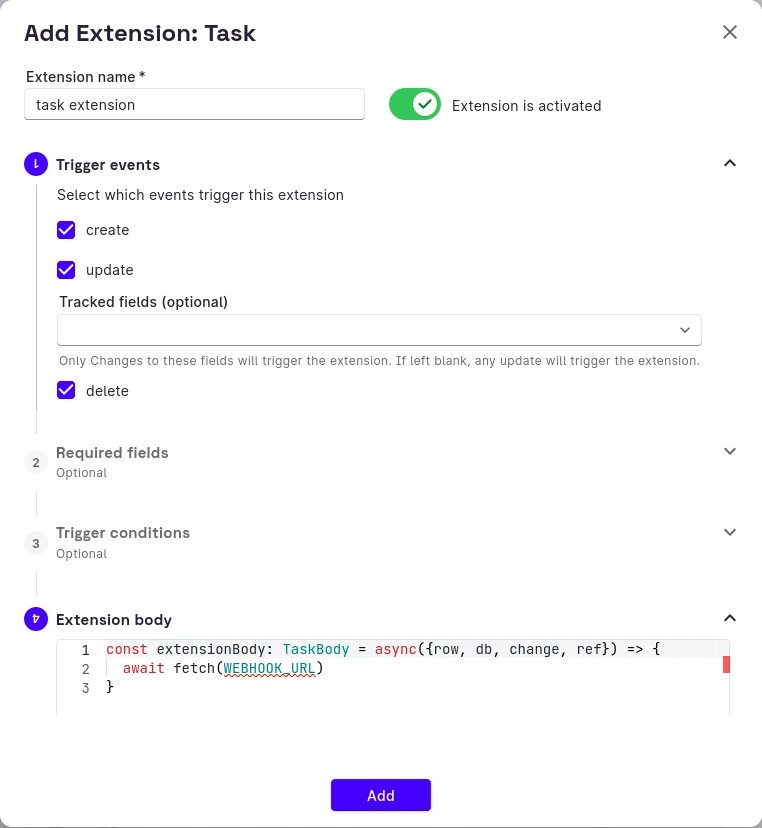# 8 Common Excel Functions In Rowy (2023): Definitions & Low-Code Examples

Office workers spend 38% of their job hours using Excel spreadsheets on average, but 12% of spreadsheets contain business-critical errors, and surveyed workers need help from colleagues regarding an Excel issue about twice a week, with the average issue taking 10 minutes to fix on average: when you need to scale your operations to more than one person and can't afford mistakes, you need to switch to a database.

The good news is, the switch to a database doesn't have to be painful: Rowy is a low-code database you can set up in minutes, with a familiar spreadsheet-like user interface. To make the transition even easier, we will go over 8 common Excel functions and how to use them in Rowy in the following article. Without further ado, let's get started!

### 1. SUM

In Excel, the SUM function adds up a range of numbers. It takes one or more arguments, which can be individual values, cell references, or ranges of cells, and returns the sum of those values. For example, to add up the values in cells A1 to A5:

``````=SUM(A1:A5)
``````

In Rowy, there is no notion of cells: each row is a document, and each column is a document field. When you need to perform an aggregate function on several rows, like SUM, you need to retrieve the rows first. For example, a spreadsheet containing country data would look like this in Rowy:To do this, the easiest way is to have a separate table to store the aggregated result. You can then use Rowy's webhook feature to automatically update the aggregated table whenever a row is updated to the original table. For example, you can create a new table called `countryMetrics` with `metricName` and `metricValue` columns, then have a basic webhook compute the SUM of inhabitants:

``````const basicParser: Parser = async({req, db, ref}) => {
const q = await db.collection("countries").get();
const countries = q.docs;

let sum = 0

countries.forEach((country) => {
sum += country.data().inhabitants;
})

ref.update({
totalInhabitants: sum
})

return true;
}
``````

In the original table, just create a Task Extension to trigger the webhook whenever a row is updated via HTTP request:### 2. AVERAGE

The AVERAGE function calculates the arithmetic mean of a range of numbers:

``````=AVERAGE(A1:A5)
``````

AVERAGE is also an aggregate function, so you can use the same method as SUM to compute the average of a column in Rowy. For example, you'll need the following webhook to compute the average of the `inhabitants` column in the `countries` table:

``````const basicParser: Parser = async({req, db, ref}) => {
const q = await db.collection("countries").get();
const countries = q.docs;

let sum = 0

countries.forEach((country) => {
sum += country.data().inhabitants;
})

ref.update({
averageInhabitants: sum / countries.length
})

return true;
}
``````

You just need to divide the sum by the number of countries to get the average.

### 3. COUNT

The COUNT function counts the number of cells in a range:

``````=COUNT(A1:A5)
``````

COUNT is another aggregate function, but Firebase provides a built-in function to count the number of documents in a collection, so you can use it directly in Rowy:

``````const basicParser: Parser = async({req, db, ref}) => {
const snapshot = await getCountFromServer(db.collection('countries'))

const count = snapshot.data().count;

ref.update({
totalCount: count
})

return true;
}
``````

Alternatively, you can also use filters to count the number of documents that match a certain condition:

``````const snapshot = await getCountFromServer(query(collectionGroupRef, where('price', <, 20.00)));
``````

This built-in function is better than retrieving all documents and counting them in the webhook, because it's counted in Firebase as a single operation, which is much faster and cheaper.

### 4. IF

IF is a logical function to test a condition. It takes three arguments: the conditional test, the value to return if the test is true, and the value to return if the test is false. For example, suppose you have a column of grades in column A, and you want to assign a letter grade based on the value of each grade. You could use the following formula in cell B1 to do this:

``````=IF(A1>=90, "A", IF(A1>=80, "B", IF(A1>=70, "C", IF(A1>=60, "D", "F"))))
``````

If the value in cell A1 is greater than or equal to 90, the formula returns "A". If the value is less than 90 but greater than or equal to 80, the formula returns "B", and so on.

IF is often used for conditional rendering: if a column has a certain value, display the matching value in the other column. In Rowy, you can use the Formula column type to achieve this:

``````const formula:Formula = async ({ row })=> {
const sum = row.subject1 + row.subject2 + row.subject3
const avg = sum/3;

if(avg>=90){
return "A"
}
else if(avg>=80 && avg<90){
return "B"
}
else if(avg>=70 && avg<80){
return "C"
}
else if(avg>=60 && avg<70){
return "D"
}
else if(avg>=50 && avg<60){
return "D"
}
else{
return "F"
}
}
``````

### 5. TRIM

TRIM removes extra spaces from a text string, except for a single space between words. This can be helpful when working with data that has inconsistent spacing, such as imported or copied text from external sources:

``````=TRIM("  Hello, world!  ")
``````

With Rowy, you can use a Derivative column to perform operations on dependent fields. For example, you can have a `title` column and a `cleanedTitle` column that removes extra spaces from the `title` column:

``````const derivative:Derivative = async ({ row })=> {
return row.title.trim()
}
``````

Though this is possible, a better approach would be to clean the data while importing it in Rowy or before processing it in another function, to avoid extra columns.

### 6. MAX & MIN

The MAX and MIN functions return the maximum and minimum value from a range of cells or values: `=MAX(A1:A5)` would return the highest value in cells A1 to A5, while `=MIN(B1:B10)` returns the lowest value in cells B1 to B10.

Max / min are also aggregate functions, so we use the same webhook method:

``````const basicParser: Parser = async({req, db, ref}) => {
const q = await db.collection("countries").get();
const countries = q.docs;

let max = 0
let min = 0

countries.forEach((country) => {
if(country.data().inhabitants > max){
max = country.data().inhabitants
}
if(country.data().inhabitants < min){
min = country.data().inhabitants
}
})

ref.update({
maxInhabitants: max,
minInhabitants: min
})

return true;
}
``````

### 7. LEN

LEN returns the length of a text string (the number of characters in it) or array (the number of elements it contains). For example, `=LEN("Hello, world!")` returns 13.

LEN is a basic Javascript function, so you can use it directly in Rowy, just like TRIM:

``````const derivative:Derivative = async ({ row })=> {
return row.title.length
}
``````

### 8. CONCATENATE

The CONCATENATE function joins two or more text strings into a single string:

``````=CONCATENATE("Hello, ", "world!")
``````

It's useful when you have for example a `firstName` and a `lastName` in separate columns, and you want to combine them into a single `fullname` column:

``````const derivative:Derivative = async ({ row })=> {
return row.firstName.trim() + ' ' + row.lastName.trim()
}
``````

## Join Rowy

Excel powers a lot of business processes, and it's a great tool for data analysis. But it's not always the best tool. If you're looking for a more powerful alternative, Rowy is a great choice. It's a spreadsheet that's built to do more with your data, and it's free to use.

If you liked this article, you'll probably enjoy our Discord community as well. We help each other make things with less code, and we're always happy to welcome new members!